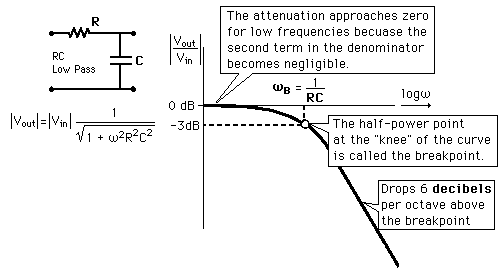# Help me understand an old project structure

Hi all!
I started using Arduino 2 years ago when I got one for a birthday. I didn't know anything about electronics nor programming but I managed (with tons of online/offline help) to create a project that checks 4 Temp LM35 sensors and open/close 4 relays which control heatpads.
After I finished that, I wanted to scale things up but I got fired from my old job and everything became a vortex so I stopped using Arduino.

Now I got a new IT job and i'm also using some of my time to learn programming (in powershell, but logic is logic) and i'm trying to get back to microcontrollers.
The thing is I forgot almost everything about Arduino, and although i'm almost done understanding the code I wrote back then (and now I know how to properly comment code to prevent this from happening again and i'm doing it), i'm having a lot of trouble understanding how I wired everything since I have 0 experience in Electronics.

There are a couple of things I want to understand that maybe you can help me with:

The project is made of 1 Arduino Uno, 1 Breadboard, 1 YwRobot PSU, 1 4-Relay Module, 4 LM35 Temp Sensors, 4 capacitors and 4 resistors.

Here's the code:

``````const byte tempPin[] = {A1, A2, A5, A4}; //defining the LM35 temp sensors input pins as constant byte to avoid changing it. Value from 0 to 255
const byte relayPin[] = {6, 7, 8, 9}; //defining the relay output pins as constant byte to avoid chaging it. Value from 0 to 255

// hysteresis = upperLimit - lowerLimit
const byte lowerLimit = 28;
const byte upperLimit = 32;

float tempC; //defining the 4 temp inputs inside an array. Float to deal with decimals
const int numReadings = 25; // defining the number of readings to create an average with
word printInterval = 1000; // 1 second
unsigned long lastPrintTime = 0;

void setup()
{
analogReference(INTERNAL); //Using the internal 1.1v reference
Serial.begin(115200); //baud speed set to max 115200
for (int i = 0; i < 4; i++) {
pinMode(relayPin[i], INPUT_PULLUP); //this sets the internal arduino 5v live as the reference to avoid the floating state of the relay digital pins when the switch is open. I need to better understand this.
pinMode(relayPin[i], OUTPUT); // defaults HIGH, relay OFF
}
}

void loop()
{
for (int i = 0; i < 4; i++) {
float raw_temp = analogRead(tempPin[i]) / 9.31;
tempC[i] += (raw_temp - tempC[i])  / numReadings;
if (tempC[i] < lowerLimit) {
digitalWrite(relayPin[i], LOW);   //relay OFF
}
else if (tempC[i] > upperLimit) {
digitalWrite(relayPin[i], HIGH);  // relay ON
}
}

if (millis() - lastPrintTime >= printInterval) {
for (int i = 0; i < 4; i++) {
Serial.print("tempC");
Serial.print(i + 1);
Serial.print(" ");
Serial.println(tempC[i]);
}
Serial.println();
lastPrintTime = millis(); // reset print timer
}
}
``````

So, here are my questions
Why is the 1.1v internal reference more accurate than the standard 5v one for reading temps? I understand I did it because it's the best way to get better readings, but I don't understand why.

Why did I connect a resistor and a capacitor between the LM35 output and the LM35 ground wire?
I think I did it to avoid fluctuations, but how does this work? or should I say why does this work?

Why is the 1.1v internal reference more accurate than the standard 5v one for reading temps?

Data sheets of LM35 have described the response of LM35 as:
When ambient Temp is 250C, the LM35 generates 25 mV signal
When ambient Temp is 1500C, the LM35 generates 150 mV signal.
The sensor is linear with some gain but no offset.

Assume that the ambient temp is 250C. Let us digitize the corresponding 25 mV signal using the 10-bit ADC of the UNO with VREF(Full Scale) at 5V. The ADC value will be: (11 1111 1111/5(Full Scale))* 0.025 = 00 0000 0101. Because of electronic noise the LS-bit could become 0 or 1; so, in the worst case, we are loosing (25/5(ADC value))1 = 5 mV signal which is 20% error (5/25100).

What will happen, if we use VREF(Full Scale) at 1.1V?
The ADC value will be: (11 1111 1111/1.1(Full Scale))* 0.025 = 00 0001 0111. Because of electronic noise the LS-bit could become 0 or 1; so, in the worst case, we are loosing (25/17(ADC value))1 = 1.5 mV signal which is 6% error (1.5/25100).

Do we want to reduce the error more? It is possible by feeding the VREF voltage from external source using AREF pin of the ATmega328P MCU.

I hope, many more better satisfying opinions will be posted in this thread on this issue.

The internal 1.1V reference is not more =accurate=, it is more =stable=. So you should calibrate it, but then it remains very close to the same voltage. In fact, it is listed in the datasheet as being 1.1V +-0.1V. But being what is called a bandgap reference, it should be very stable over time and temperature.Once, while trying to tame a bad case of LM35 jitters, after trying caps from inPin to GND, AREF pin to GND, various Rs from inPin to GND, before giving up I stuck a 1k R in series between LM35 out and inPin (A0), instantly, the numbers became rock steady, not even 1 count of back and forth dither. But didn't work with other LM35s or TMP36s, so? Don't axe me.